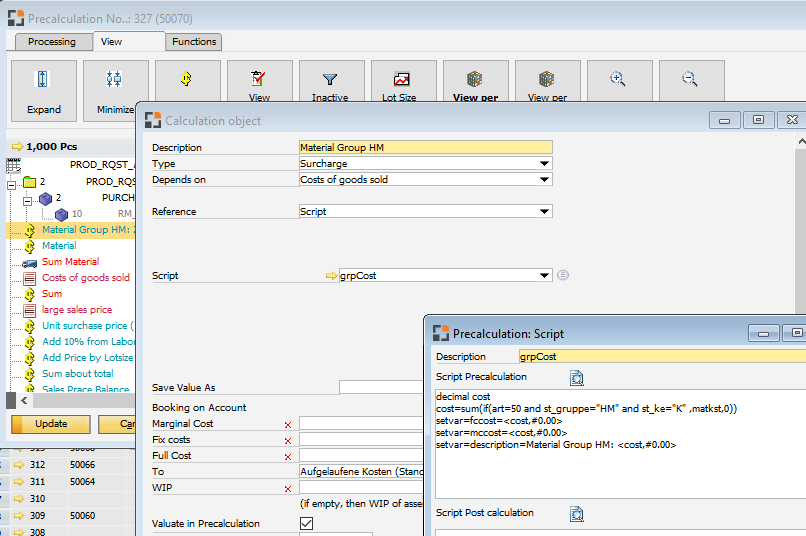Skip to main content

## Comments

3 comments

•You can create own schema objects with scriptnow you can sum all material group positions (more see documentation (F1 in this window))

Material line art=50
Purchase item: st_ke='E'
Price is in matkst

Problem: if you work with for - next, this can be slow

follow idea:

this is a datawindow, which support expression (see appeon help pages)

in beas you can call expression,if you use decimal values

instance decimal lc_value

all formulas evualated in dw_1. Note: this is not working in batch calculation, because here we use "dw_2"

formula

lc_value=sum(if(art=50 and st_gruppe="HM" ,matkst,0))

and then you can return the result

setvar=fccost=<cost,#0.00>

Note: don't forget the correct conversion with "#0.00"

complete script is

decimal cost
cost=sum(if(art=50 and st_gruppe="HM" and st_ke="K" ,matkst,0))
setvar=fccost=<cost,#0.00>
setvar=mccost=<cost,#0.00>
setvar=description=Material Group HM: <cost,#0.00>

Note:

Not all rules included. No quarantee that it's working in every environment. Not working in batch calculation or post calculation

•Good morning, I tried to filter my group but it doesn't work, the type filter works.
I left it on the filter until the group field alone to test but nothing unfortunately.

Test1 - failed

decimal cost
cost=sum(if(st_gruppe="102" ,matkst,0))
setvar=fccost=<cost,#0.0000>
setvar=mccost=<cost,#0.0000>
setvar=description=Material Intermediário: <cost,#0.0000>

Test 2  - failed

decimal cost
cost=sum(if(art=50 and st_gruppe="102" and st_ke="K" ,matkst,0))
setvar=fccost=<cost,#0.00>
setvar=mccost=<cost,#0.00>
setvar=description=Material Intermediário: <cost,#0.00>

Test 3 - Pass

decimal cost
cost=sum(if(art=50 and st_ke="E" ,matkst,0))
setvar=fccost=<cost,#0.0000>
setvar=mccost=<cost,#0.0000>
setvar=description=Material Intermediário: <cost,#0.0000>

In F1 there is no explanation of these variables that were used in the example.

Regards

Fabio.

•Hi Fabio, i know. This solution is only  a trick and is not working in every environment or batch calculation. This is the reason that we didn't described it

But if you can live with this limitations, then it's a fast good solution

Note

st_ke="E" = you make a sum of all assemblies
st_ke="K" = purchage items

Please sign in to leave a comment.